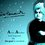# INDIAN NATIONAL PHYSICS OLYMPIAD - 1998 QUESTION

1) A merry go-round rotates with a constant angular speed $\omega$. Relative to the rotating frame of the merry go-round, an outside object(say a man) stationary on the ground appears to revolve with the same angular speed $\omega$ in the opposite sense. Explain the motion of the object in terms of pseudo-forces acting on it in the rotating frame of reference.

Do the complete Vector analysis

2) Show that is a collision is to take place between a fast moving electron and an electron at rest is to lead to production of an electron-positron pair, the K.E of the fast electron should be atleast $.6m{ c }^{ 2 }$, where m is the mass of electron and c is the speed of light.Note by Tushar Gopalka
6 years, 6 months ago

This discussion board is a place to discuss our Daily Challenges and the math and science related to those challenges. Explanations are more than just a solution — they should explain the steps and thinking strategies that you used to obtain the solution. Comments should further the discussion of math and science.

When posting on Brilliant:

• Use the emojis to react to an explanation, whether you're congratulating a job well done , or just really confused .
• Ask specific questions about the challenge or the steps in somebody's explanation. Well-posed questions can add a lot to the discussion, but posting "I don't understand!" doesn't help anyone.
• Try to contribute something new to the discussion, whether it is an extension, generalization or other idea related to the challenge.

MarkdownAppears as
*italics* or _italics_ italics
**bold** or __bold__ bold
- bulleted- list
• bulleted
• list
1. numbered2. list
1. numbered
2. list
Note: you must add a full line of space before and after lists for them to show up correctly
paragraph 1paragraph 2

paragraph 1

paragraph 2

[example link](https://brilliant.org)example link
> This is a quote
This is a quote
    # I indented these lines
# 4 spaces, and now they show
# up as a code block.

print "hello world"
# I indented these lines
# 4 spaces, and now they show
# up as a code block.

print "hello world"
MathAppears as
Remember to wrap math in $$ ... $$ or $ ... $ to ensure proper formatting.
2 \times 3 $2 \times 3$
2^{34} $2^{34}$
a_{i-1} $a_{i-1}$
\frac{2}{3} $\frac{2}{3}$
\sqrt{2} $\sqrt{2}$
\sum_{i=1}^3 $\sum_{i=1}^3$
\sin \theta $\sin \theta$
\boxed{123} $\boxed{123}$

Sort by:

Yep, @Tushar Gopalka solved, actually question meant that due to the collision, the energy released should be converted to positron and electron , the preexisting electrons are still present, hence charge conserved, heres the solution.,

Let the initial spped be u, corresponding gamma factor is $\gamma$ , and mass of electron 'm', we wish that it should create a pair,, so on RHS, we have 3 electrons and one posistron, each has mass 'm',

for min energy, we want maximum inelasticity in colision so that most energy is lost by the 1st electron and this is used for pair creation, hence we want all 4 particles to move in same line after collision,,

Let them move with velocity 'v' and have gamma factor $\alpha =\frac { 1 }{ \sqrt { 1-{ v }^{ 2 } } }$

hence, conserving momentum and energy (note however that in relativity, momentum is given as $\gamma mv$ and energy as $\gamma m{ c }^{ 2 }$ )

*also i take c=1 , for convenient calulation *

now here we go,

$\gamma m+m=4\alpha m$

also

$\gamma mv\quad =4mv\alpha$

dividing both

$v=\frac { \gamma }{ \gamma +1 } u$

substituting this in 2nd equation and using $\varepsilon =\frac { 1 }{ \sqrt { 1-{ v }^{ 2 } } }$

we have

$16=\frac { 1-{ u }^{ 2 } }{ 1-{ u }^{ 2 } } +2\gamma +1=2+2\gamma \quad or\quad \gamma =7\\ \\ hence\quad KE\quad =\quad (\gamma m-m){ c }^{ 2 }=6m{ c }^{ 2 }\quad$

hence proved

- 6 years, 6 months ago

Yeah ,, see using concept of pseudoforces,, the forces on the man are centripetal and coriolis forces,, now the centripetal force acts radially outward (since the corresponding acceleration is radially inward) and it has magnitude mw(^2)R

, now the coriolis force is is given by -2m(w x v ) (where x means cross product) , you can check that it acts inward and it has magnitude 2mw(^2)R , hence net force is inward which is mw(^2)R and hence the man rotates in a circle ,,,

It is in opposite sense which is fairly obvious due to relative velocity

i shall solve the second one and report back , but you are sure that is the exact wording of the problem? because charge must be conserved,

also can you please provide me the source to those INPHO papers,, i only got recent ones from net

- 6 years, 6 months ago

You have to buy it from hbcse website. But it takes two months to get the delivery

- 6 years, 6 months ago

got it, on it, if i succeed, i shall tell

- 6 years, 6 months ago

Yes the wording is correct.

- 6 years, 6 months ago